Theory and Modern Applications

The growth of solutions of systems of complex q-shift difference equations

Abstract

In this paper, we study the properties of systems of two types of complex q-shift difference equations with meromorphic solutions from the point of view of Nevanlinna theory. Some results obtained in this paper improve and extend the previous theorems given by Gao, and five examples show the extension of solutions of the system of complex difference equation.

MSC:39A50, 30D35.

1 Introduction and main results

In this paper, the problem of the existence and growth of solutions of complex difference equations will be studied. The fundamental results and the standard notations of the Nevanlinna value distribution theory of meromorphic functions will be used in this paper (see ). In addition, for a meromorphic function f, $S\left(r,f\right)$ denotes any quantity satisfying $S\left(r,f\right)=o\left(T\left(r,f\right)\right)$ for all r outside of a possible exceptional set E of finite logarithmic measure ${lim}_{r\to \mathrm{\infty }}{\int }_{\left[1,r\right)\cap E}\frac{dt}{t}<\mathrm{\infty }$, ${S}_{1}\left(r,f\right)$ denotes any quantity satisfying ${S}_{1}\left(r,f\right)=o\left(T\left(r,f\right)\right)$ for all r on a set F of logarithmic density 1 (or for all r outside of a possible exceptional set ${F}^{\prime }$ of logarithmic density 0, the logarithmic density of a set F is defined by

$\underset{r\to \mathrm{\infty }}{lim sup}\frac{1}{logr}{\int }_{\left[1,r\right]\cap F}\frac{1}{t}\phantom{\rule{0.2em}{0ex}}dt.$

Throughout this paper, the set E of finite logarithmic measure and F of logarithmic density will be not necessarily the same at each occurrence.

In recent years, there has been an increasing interest in studying difference equations, difference product and q-difference in the complex plane , considerable attention was paid to the growth of solutions of difference equations, value distribution and the uniqueness of differences analogues of Nevanlinna’s theory . Chiang and Feng , Halburd and Korhonen  established a difference analogue of the logarithmic derivative lemma independently, and Barnett, Halburd, Korhonen and Morgan  also established an analogue of the logarithmic derivative lemma on q-difference operators. After their works, many people applied it to prove a number of results on meromorphic solutions of complex difference equations.

In 2000, Ablowitz, Halburd and Herbst  studied some class of complex difference equations,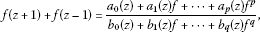(1)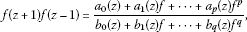(2)

where the coefficients are meromorphic functions, and obtained the following results.

Theorem 1.1 (see )

If the difference equation (1) (or (2)) with polynomial coefficients ${a}_{i}\left(z\right)$, ${b}_{i}\left(z\right)$ admits a transcendental meromorphic solution of finite order, then $d=max\left\{p,q\right\}\le 2$.

In 2001, Heittokangas et al.  further investigated some complex difference equations which are similar to (1) and (2) and obtained the following results which are the improvement of Theorems 1.1 and 1.2.

Theorem 1.2 (see [, Proposition 8 and Proposition 9])

Let ${c}_{1},\dots ,{c}_{n}\in \mathbb{C}\setminus \left\{0\right\}$. If the equations

$\begin{array}{c}\sum _{i=1}^{n}f\left(z+{c}_{j}\right)=R\left(z,f\left(z\right)\right),\phantom{\rule{2em}{0ex}}\prod _{i=1}^{n}f\left(z+{c}_{j}\right)=R\left(z,f\left(z\right)\right),\hfill \\ R\left(z,f\left(z\right)\right):=\frac{P\left(z,f\left(z\right)\right)}{Q\left(z,f\left(z\right)\right)}=\frac{{a}_{0}\left(z\right)+{a}_{1}\left(z\right)f+\cdots +{a}_{s}\left(z\right){f}^{s}}{{b}_{0}\left(z\right)+{b}_{1}\left(z\right)f+\cdots +{b}_{t}\left(z\right){f}^{t}}\hfill \end{array}$

with rational coefficients ${a}_{i}\left(z\right)$, ${b}_{i}\left(z\right)$ admit a transcendental meromorphic solution of finite order, then $d=max\left\{s,t\right\}$.

In 2002, Gundersen et al.  studied the growth of meromorphic solutions of q-difference equations and obtained the result as follows.

Theorem 1.3 (see [, Theorem 3.2])

Suppose that f is a transcendental meromorphic solution of an equation of the form

$f\left(cz\right)=R\left(z,f\left(z\right)\right)=\frac{{\sum }_{i=0}^{s}{a}_{i}\left(z\right)f{\left(z\right)}^{i}}{{\sum }_{j=0}^{t}{b}_{j}\left(z\right)f{\left(z\right)}^{j}}$

with meromorphic coefficients ${a}_{i}\left(z\right)$, ${b}_{j}\left(z\right)$ and a constant $c\ne 0$ ($|c|>1$), assuming that $d:=max\left\{s,t\right\}\ge 1$, ${a}_{s}\left(z\right)\ne 0$, ${b}_{t}\left(z\right)\ne 0$, and that $R\left(z,f\left(z\right)\right)$ is irreducible in f. Then $\rho \left(f\right)=\frac{logd}{log|c|}$.

In 2010, Zheng and Chen  further considered the growth of meromorphic solutions of q-difference equations and obtained some results which extended the theorems given by Heittokangas et al. .

Theorem 1.4 (see )

Suppose that f is a transcendental meromorphic solution of the equation of the form

$\frac{{\sum }_{\lambda \in I}{d}_{\lambda }\left(z\right)f{\left(qz\right)}^{{i}_{{\lambda }_{1}}}f{\left({q}^{2}z\right)}^{{i}_{{\lambda }_{2}}}\cdots f{\left({q}^{n}z\right)}^{{i}_{{\lambda }_{n}}}}{{\sum }_{\mu \in J}{e}_{\mu }\left(z\right)f{\left(qz\right)}^{{j}_{{\mu }_{1}}}f{\left({q}^{2}z\right)}^{{j}_{{\mu }_{2}}}\cdots f{\left({q}^{n}z\right)}^{{j}_{{\mu }_{n}}}}=R\left(z,f\left(z\right)\right),$
(3)

where $I=\left\{\left({i}_{{\lambda }_{1}},{i}_{{\lambda }_{2}},\dots ,{i}_{{\lambda }_{n}}\right)\right\}$, $J=\left\{{j}_{{\mu }_{1}},{j}_{{\mu }_{2}},\dots ,{j}_{{\mu }_{n}}\right\}$ are two finite index sets satisfying

$\underset{\lambda ,\mu }{max}\left\{{i}_{{\lambda }_{1}}+{i}_{{\lambda }_{2}}+\cdots +{i}_{{\lambda }_{n}},{j}_{{\mu }_{1}}+{j}_{{\mu }_{2}}+\cdots +{j}_{{\mu }_{n}}\right\}=\sigma ,$

$q\in \mathbb{C}\setminus \left\{0\right\}$, $|q|>1$, $d\ge 2$, and all coefficients of (3) are of growth $S\left(r,f\right)$. If $d=max\left\{s,t\right\}>2n\sigma$, then for sufficiently large r, $T\left(r,f\right)\ge K{\left(\frac{d}{2n\sigma }\right)}^{\frac{logr}{nlog|q|}}$, where K (>0) is a constant. Thus, the lower order of f satisfies $\mu \left(f\right)\ge \frac{logd-log2n\sigma }{nlog|q|}$.

In 2011, Liu and Qi  investigated the properties of meromorphic solutions of a q-shift difference equation and obtained some results as follows.

Theorem 1.5 (see [, Theorem 4.1])

Suppose that f is a transcendental meromorphic solution of

$f\left(cz+\eta \right)=R\left(z,f\left(z\right)\right)=\frac{{\sum }_{i=0}^{s}{a}_{i}\left(z\right)f{\left(z\right)}^{i}}{{\sum }_{j=0}^{t}{b}_{j}\left(z\right)f{\left(z\right)}^{j}},$

where the coefficients ${a}_{i}\left(z\right)$, ${b}_{j}\left(z\right)$ are rational, and $|c|>1$. Assuming $R\left(z,f\left(z\right)\right)$ is irreducible in f, ${a}_{s}\left(z\right){b}_{t}\left(z\right)\not\equiv 0$. If $s>t+1$ and $m:=s-t$, then $\lambda \left(1/f\right)\ge log|m|/log|c|$, provided that f has infinitely many poles.

In 2012, Gao  investigated a complex difference equation which is similar to (3) and obtained the following theorem.

Theorem 1.6 (see [, Theorem 1.1])

Let ${c}_{1},\dots ,{c}_{n}\in \mathbb{C}\setminus \left\{0\right\}$. If the equation

$\frac{{\sum }_{\lambda \in I}{d}_{\lambda }\left(z\right)f{\left(z\right)}^{{i}_{{\lambda }_{0}}}f{\left(z+{c}_{1}\right)}^{{i}_{{\lambda }_{1}}}f{\left(z+{c}_{2}\right)}^{{i}_{{\lambda }_{2}}}\cdots f{\left(z+{c}_{n}\right)}^{{i}_{{\lambda }_{n}}}}{{\sum }_{\mu \in J}{e}_{\mu }\left(z\right)f{\left(z\right)}^{{j}_{{\mu }_{0}}}f{\left(z+{c}_{1}\right)}^{{j}_{{\mu }_{1}}}f{\left(z+{c}_{2}\right)}^{{j}_{{\mu }_{2}}}\cdots f{\left(z+{c}_{n}\right)}^{{j}_{{\mu }_{n}}}}=R\left(z,f\left(z\right)\right)$
(4)

has a transcendental meromorphic solution of finite order, then

$max\left\{s,t\right\}\le {\mathrm{\Gamma }}_{1}+{\mathrm{\Gamma }}_{2},$

where ${\mathrm{\Gamma }}_{1}=max\left\{{i}_{{\lambda }_{0}}+{i}_{{\lambda }_{1}}+\cdots +{i}_{{\lambda }_{n}}\right\}$, ${\mathrm{\Gamma }}_{2}=max\left\{{j}_{{\mu }_{0}}+{j}_{{\mu }_{1}}+\cdots +{j}_{{\mu }_{n}}\right\}$, ${d}_{\lambda }\left(z\right)$, ${e}_{\mu }\left(z\right)$, ${a}_{j}\left(z\right)$ and ${b}_{j}\left(z\right)$ are small functions of f.

Thus, the following questions arise naturally:

Question A Will the assertion of Theorem 1.6 remain valid if the transcendental meromorphic solution has infinite order?

Question B What will happen when $f\left(z+{c}_{i}\right)$ is replaced by $f\left({q}^{i}z+{c}_{i}\right)$ for $i=1,2,\dots ,n$ in Theorem 1.6?

In this paper, we investigate the above questions and obtain the following theorem.

Theorem 1.7 Let $q,{c}_{1},\dots ,{c}_{n}\in \mathbb{C}\setminus \left\{0\right\}$, and ${d}_{\lambda }\left(z\right)$, ${e}_{\mu }\left(z\right)$, ${a}_{i}\left(z\right)$ and ${b}_{j}\left(z\right)$ be of growth $o\left(T\left(r,f\right)\right)$ without an exceptional set as $r\to \mathrm{\infty }$. If f is a transcendental meromorphic solution of the equation of the form

$\frac{{\sum }_{\lambda \in I}{d}_{\lambda }\left(z\right)f{\left(z\right)}^{{i}_{{\lambda }_{0}}}f{\left(qz+{c}_{1}\right)}^{{i}_{{\lambda }_{1}}}f{\left({q}^{2}z+{c}_{2}\right)}^{{i}_{{\lambda }_{2}}}\cdots f{\left({q}^{n}z+{c}_{n}\right)}^{{i}_{{\lambda }_{n}}}}{{\sum }_{\mu \in J}{e}_{\mu }\left(z\right)f{\left(z\right)}^{{i}_{{\lambda }_{0}}}f{\left(qz+{c}_{1}\right)}^{{j}_{{\mu }_{1}}}f{\left({q}^{2}z+{c}_{2}\right)}^{{j}_{{\mu }_{2}}}\cdots f{\left({q}^{n}z+{c}_{n}\right)}^{{j}_{{\mu }_{n}}}}=R\left(z,f\left(z\right)\right)$
(5)

and satisfies one of the following conditions:

1. (i)

$q=1$ and ${\rho }_{2}\left(f\right)<1$;

2. (ii)

$q\ne 1$ and $\rho \left(f\right)=0$.

Then the conclusion of Theorem  1.6 still holds.

Remark 1.1 Theorem 1.7 is an improvement of Theorem 1.6. And Theorem 1.7 is an answer to Question A and Question B.

In this paper, we also investigated the extension of a meromorphic solution of the following system of complex q-shift difference equations of the form:

$\left\{\begin{array}{c}{P}_{1}\left(z,{f}_{1}\left(qz+\eta \right)\right)={\sum }_{{J}_{1}}{a}_{{J}_{1}}\left(z\right)\left({\prod }_{j\in {J}_{1}}{f}_{2}\left(z+{c}_{j}\right)\right),\hfill \\ {P}_{2}\left(z,{f}_{2}\left(qz+\eta \right)\right)={\sum }_{{J}_{2}}{a}_{{J}_{2}}\left(z\right)\left({\prod }_{j\in {J}_{2}}{f}_{1}\left(z+{c}_{j}\right)\right),\hfill \end{array}$
(6)

and

$\left\{\begin{array}{c}{\mathrm{\Omega }}_{1}\left(z,{f}_{1},{f}_{2}\right)={R}_{1}\left(z,{f}_{1}\right),\hfill \\ {\mathrm{\Omega }}_{2}\left(z,{f}_{1},{f}_{2}\right)={R}_{2}\left(z,{f}_{2}\right),\hfill \end{array}$
(7)

where $q,\eta ,{c}_{1},\dots ,{c}_{n}\in \mathbb{C}\setminus \left\{0\right\}$, $\left\{{J}_{\iota }\right\}$ is a collection of all subsects of $\left\{1,2,\dots ,{n}_{\iota }\right\}$, $\iota =1,2$, two q-shift difference polynomials ${\mathrm{\Omega }}_{1}\left(z,{f}_{1},{f}_{2}\right)$, ${\mathrm{\Omega }}_{2}\left(z,{f}_{1},{f}_{2}\right)$ are defined by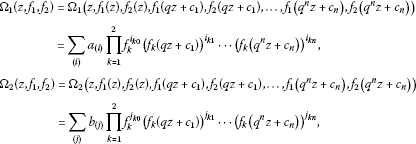and ${R}_{1}\left(z,{f}_{1}\right)$, ${R}_{2}\left(z,{f}_{2}\right)$ are defined by

$\begin{array}{c}{R}_{1}\left(z,{f}_{1}\right)=\frac{{P}_{1}\left(z,{f}_{1}\right)}{{Q}_{1}\left(z,{f}_{1}\right)}=\frac{{\sum }_{i=0}^{{s}_{1}}{a}_{i}^{1}\left(z\right){f}_{1}^{i}}{{\sum }_{j=0}^{{t}_{1}}{b}_{j}^{1}\left(z\right){f}_{1}^{j}},\hfill \\ {R}_{2}\left(z,{f}_{2}\right)=\frac{{P}_{2}\left(z,{f}_{2}\right)}{{Q}_{2}\left(z,{f}_{2}\right)}=\frac{{\sum }_{i=0}^{{s}_{2}}{a}_{i}^{2}\left(z\right){f}_{2}^{i}}{{\sum }_{j=0}^{{t}_{2}}{b}_{j}^{2}\left(z\right){f}_{2}^{j}},\hfill \end{array}$

and all coefficients $\left\{{a}_{i}^{1}\left(z\right)\right\}$, $\left\{{a}_{i}^{2}\left(z\right)\right\}$, $\left\{{b}_{i}^{1}\left(z\right)\right\}$, $\left\{{b}_{i}^{2}\left(z\right)\right\}$, $\left\{{a}_{\left(i\right)}\left(z\right)\right\}$, $\left\{{b}_{\left(i\right)}\left(z\right)\right\}$ and ${a}_{{J}_{1}}\left(z\right)$, ${a}_{{J}_{2}}\left(z\right)$ are small functions of ${f}_{1}$, ${f}_{2}$, and ${a}_{{s}_{l}}^{l}{b}_{{t}_{l}}^{l}\ne 0$ for $l=1,2$. Let

$\begin{array}{c}{\mathrm{\Gamma }}_{11}=\underset{\left(i\right)}{max}\left\{\sum _{\nu =0}^{n}{i}_{1\nu }\right\},\phantom{\rule{2em}{0ex}}{\mathrm{\Gamma }}_{12}=\underset{\left(i\right)}{max}\left\{\sum _{\nu =0}^{n}{i}_{2\nu }\right\},\hfill \\ {\mathrm{\Gamma }}_{21}=\underset{\left(j\right)}{max}\left\{\sum _{\nu =0}^{n}{j}_{1\nu }\right\},\phantom{\rule{2em}{0ex}}{\mathrm{\Gamma }}_{22}=\underset{\left(j\right)}{max}\left\{\sum _{\nu =0}^{n}{j}_{2\nu }\right\},\hfill \end{array}$

and

$S\left(r\right):=\sum _{l=1}^{2}\left(\sum T\left(r,{a}_{i}^{l}\right)+\sum T\left(r,{b}_{j}^{l}\right)\right)+\sum _{\left(i\right)}T\left(r,{a}_{\left(i\right)}\right)+\sum _{\left(j\right)}T\left(r,{b}_{\left(j\right)}\right).$

The order and hyper order of a meromorphic solution $\left({f}_{1},{f}_{2}\right)$ of the system (6) are defined by

$\rho =\rho \left({f}_{1},{f}_{2}\right)=max\left\{\rho \left({f}_{1}\right),\rho \left({f}_{2}\right)\right\},\phantom{\rule{2em}{0ex}}{\rho }_{2}={\rho }_{2}\left({f}_{1},{f}_{2}\right)=max\left\{{\rho }_{2}\left({f}_{1}\right),{\rho }_{2}\left({f}_{2}\right)\right\},$

where

$\rho \left({f}_{k}\right)=\underset{r\to \mathrm{\infty }}{lim sup}\frac{logT\left(r,{f}_{k}\right)}{logr},\phantom{\rule{2em}{0ex}}{\rho }_{2}\left({f}_{k}\right)=\underset{r\to \mathrm{\infty }}{lim sup}\frac{loglogT\left(r,{f}_{k}\right)}{logr},\phantom{\rule{1em}{0ex}}k=1,2.$

To state our main results, we require the following definition.

Definition 1.8 (see )

If $\left({f}_{1},{f}_{2}\right)$ is a meromorphic solution of the equation system (6), and its component ${f}_{k}$ satisfies

$\underset{r\to \mathrm{\infty },r\notin E}{lim sup}\frac{S\left(r\right)}{T\left(r,{f}_{k}\right)}=0,\phantom{\rule{1em}{0ex}}k=1,2,$

then ${f}_{k}$ is called admissible, where E is an exceptional set with finite logarithmic measure.

Theorem 1.9 For the system (6), $\left\{{J}_{i}\right\}$ are two collections of all non-empty subsets of $\left\{1,2,\dots ,{n}_{i}\right\}$ for $i=1,2$, ${c}_{j}$ ($j=1,2,\dots ,n$) are distinct complex constants, and ${P}_{i}\left(z,u\right)$ is a polynomial in u of ${deg}_{u}={s}_{i}$ (>0), its coefficients of ${P}_{i}\left(z,u\right)$ are all small functions of ${f}_{1}$${f}_{2}$. Let $\left({f}_{1},{f}_{2}\right)$ be a meromorphic solution of the system (6) such that ${f}_{1}$, ${f}_{2}$ are non-rational meromorphic, and all the coefficients of (6) are small functions relative to ${f}_{1}$, ${f}_{2}$. Thus,

1. (i)

if $0<|q|<1$ and ${s}_{1}{s}_{2}\ge {n}_{1}{n}_{2}$, we have

$\mu \left({f}_{1}\right)+\mu \left({f}_{2}\right)\ge \frac{log{s}_{1}{s}_{2}-log{n}_{1}{n}_{2}}{-log|q|};$
(8)
2. (ii)

if $|q|>1$ and ${s}_{1}{s}_{2}\le {n}_{1}{n}_{2}$, then

$\rho \left({f}_{1}\right)+\rho \left({f}_{2}\right)\le \frac{log{n}_{1}{n}_{2}-log{s}_{1}{s}_{2}}{log|q|};$
(9)
3. (iii)

if $q=1$ and ${s}_{1}{s}_{2}\ge {n}_{1}{n}_{2}$, then $\mu \left({f}_{1}\right)+\mu \left({f}_{2}\right)\ge \mathrm{\infty }$,

where $\mu \left(f\right)$ is the lower order of f.

Some examples will show that the conclusions (8) and (9) in Theorem 1.9 are sharp.

Example 1.1 The function $\left({f}_{1}\left(z\right),{f}_{2}\left(z\right)\right)=\left({e}^{z},{e}^{-z}\right)$ satisfies the system

$\left\{\begin{array}{c}{\left[{f}_{1}\left(z+c\right)\right]}^{2}{\left[{f}_{1}\left(z-c\right)\right]}^{2}+{f}_{1}\left(z+2c\right)+{f}_{1}\left(z-2c\right)\hfill \\ \phantom{\rule{1em}{0ex}}={a}_{1}\left(z\right){\left({f}_{2}\left(qz+\eta \right)\right)}^{8}+{b}_{1}\left(z\right){\left({f}_{2}\left(qz+\eta \right)\right)}^{2},\hfill \\ {}^{2}\left[{f}_{2}\left(z-c\right)\right]^{2}+{f}_{2}\left(z+2c\right)+{f}_{2}\left(z-2c\right)\hfill \\ \phantom{\rule{1em}{0ex}}={a}_{2}\left(z\right){\left({f}_{1}\left(qz+\eta \right)\right)}^{8}+{b}_{2}\left(z\right){\left({f}_{1}\left(qz+\eta \right)\right)}^{2},\hfill \end{array}$

where c, η are any nonzero complex constants, $q=-\frac{1}{2}$, and

${a}_{1}\left(z\right)={e}^{8\eta },\phantom{\rule{2em}{0ex}}{b}_{1}\left(z\right)={e}^{2\eta }\left({e}^{2c}+{e}^{-2c}\right),\phantom{\rule{2em}{0ex}}{a}_{2}\left(z\right)={e}^{-8\eta },\phantom{\rule{2em}{0ex}}{b}_{2}\left(z\right)={e}^{-2\eta }\left({e}^{2c}+{e}^{-2c}\right).$

Thus, we have

$\mu \left({f}_{1}\right)+\mu \left({f}_{2}\right)=1+1=\frac{log{s}_{1}{s}_{2}-log{n}_{1}{n}_{2}}{-log|q|},$

where ${s}_{1}={s}_{2}=8$, ${n}_{1}={n}_{2}=4$ and $|q|=\frac{1}{2}<1$. This example shows that the equality in (8) can be arrived at.

Example 1.2 The function $\left({f}_{1}\left(z\right),{f}_{2}\left(z\right)\right)=\left({e}^{{z}^{2}},{e}^{{\left(z+1\right)}^{2}}\right)$ satisfies the system

$\left\{\begin{array}{c}{f}_{1}\left(z+c\right)+{f}_{1}\left(z-c\right)={a}_{1}\left(z\right){\left({f}_{2}\left(qz+\eta \right)\right)}^{4},\hfill \\ {f}_{2}\left(z+c\right)+{f}_{2}\left(z-c\right)={a}_{2}\left(z\right){\left({f}_{1}\left(qz+\eta \right)\right)}^{4},\hfill \end{array}$

where c is any nonzero complex constant, $q=\frac{1}{2}$, $\eta =-1$, and

${a}_{1}\left(z\right)={e}^{{c}^{2}}\left({e}^{2zc}+{e}^{-2zc}\right),\phantom{\rule{2em}{0ex}}{a}_{2}\left(z\right)={e}^{{\left(c+1\right)}^{2}}\left({e}^{2z\left(c+1\right)}+{e}^{-2z\left(c+1\right)-4c}\right){e}^{4z-4}.$

We note that ${a}_{1}\left(z\right)$, ${a}_{2}\left(z\right)$ are small functions relative to ${e}^{{z}^{2}}$, ${e}^{{\left(z+1\right)}^{2}}$. Thus, we have

$\mu \left({f}_{1}\right)+\mu \left({f}_{2}\right)=4>2=\frac{log{s}_{1}{s}_{2}-log{n}_{1}{n}_{2}}{-log|q|},$

where ${s}_{1}={s}_{2}=4$, ${n}_{1}={n}_{2}=2$ and $|q|=\frac{1}{2}<1$. This example shows that the inequality (8) is true.

Example 1.3 The function $\left({f}_{1}\left(z\right),{f}_{2}\left(z\right)\right)=\left({e}^{z},{e}^{-z}\right)$ satisfies the system

$\left\{\begin{array}{c}{f}_{1}\left(z+c\right){f}_{1}\left(z-c\right){f}_{1}\left(z+2c\right){f}_{1}\left(z-2c\right)+{f}_{1}\left(z+c\right){f}_{1}\left(z-c\right)\hfill \\ \phantom{\rule{1em}{0ex}}={a}_{1}\left(z\right){\left({f}_{2}\left(qz+\eta \right)\right)}^{2}+{b}_{1}\left(z\right){f}_{2}\left(qz+\eta \right),\hfill \\ {f}_{2}\left(z+c\right){f}_{2}\left(z-c\right){f}_{2}\left(z+2c\right){f}_{2}\left(z-2c\right)+{f}_{2}\left(z+c\right){f}_{2}\left(z-c\right)\hfill \\ \phantom{\rule{1em}{0ex}}={a}_{2}\left(z\right){\left({f}_{1}\left(qz+\eta \right)\right)}^{2}+{b}_{2}\left(z\right){f}_{1}\left(qz+\eta \right),\hfill \end{array}$

where c, η are any nonzero complex constants, $q=-2$, and

${a}_{1}\left(z\right)={e}^{2\eta },\phantom{\rule{2em}{0ex}}{b}_{1}\left(z\right)={e}^{\eta },\phantom{\rule{2em}{0ex}}{a}_{2}\left(z\right)={e}^{-2\eta },\phantom{\rule{2em}{0ex}}{b}_{2}\left(z\right)={e}^{-\eta }.$

Thus, we have

$\rho \left({f}_{1}\right)+\rho \left({f}_{2}\right)=2=\frac{log{n}_{1}{n}_{2}-log{s}_{1}{s}_{2}}{log|q|},$

where ${n}_{1}={n}_{2}=4$, ${s}_{1}={s}_{2}=2$ and $|q|=2>1$. This example shows that the equality in (9) can be arrived at.

Example 1.4 The function $\left({f}_{1}\left(z\right),{f}_{2}\left(z\right)\right)=\left({e}^{{z}^{2}},{e}^{{\left(z+1\right)}^{2}}\right)$ satisfies the system

$\left\{\begin{array}{c}{d}_{1}\left(z\right){f}_{1}\left(z+c\right){f}_{1}\left(z-c\right){f}_{1}\left(z+2c\right){f}_{1}\left(z-2c\right){f}_{1}\left(z+3c\right){f}_{1}\left(z-3c\right)\hfill \\ \phantom{\rule{2em}{0ex}}×{f}_{1}\left(z+4c\right){f}_{1}\left(z-4c\right)+{d}_{2}\left(z\right){f}_{1}\left(z+c\right){f}_{1}\left(z-c\right){f}_{1}\left(z+\frac{c}{2}\right){f}_{1}\left(z-\frac{c}{2}\right)\hfill \\ \phantom{\rule{1em}{0ex}}={a}_{1}\left(z\right){\left({f}_{2}\left(qz+\eta \right)\right)}^{2}+{b}_{1}\left(z\right){f}_{2}\left(qz+\eta \right),\hfill \\ {e}_{1}\left(z\right){f}_{2}\left(z+c\right){f}_{2}\left(z-c\right){f}_{2}\left(z+2c\right){f}_{2}\left(z-2c\right){f}_{2}\left(z+3c\right){f}_{2}\left(z-3c\right)\hfill \\ \phantom{\rule{2em}{0ex}}×{f}_{2}\left(z+4c\right){f}_{2}\left(z-4c\right)+{e}_{2}\left(z\right){f}_{2}\left(z+c\right){f}_{2}\left(z-c\right){f}_{2}\left(z+\frac{c}{2}\right){f}_{2}\left(z-\frac{c}{2}\right)\hfill \\ \phantom{\rule{1em}{0ex}}={a}_{2}\left(z\right){\left({f}_{1}\left(qz+\eta \right)\right)}^{2}+{b}_{2}\left(z\right){f}_{1}\left(qz+\eta \right),\hfill \end{array}$

where c is a nonzero constant, $q=2$, $\eta =-1$,

${d}_{1}\left(z\right)={e}^{-60{c}^{2}},\phantom{\rule{2em}{0ex}}{d}_{2}\left(z\right)={e}^{-\frac{5}{2}{c}^{2}},\phantom{\rule{2em}{0ex}}{e}_{1}\left(z\right)={e}^{-60{c}^{2}-8}{e}^{-16z},\phantom{\rule{2em}{0ex}}{e}_{2}\left(z\right)={e}^{-\frac{5}{2}{c}^{2}-4}{e}^{-8z},$

and

${a}_{1}\left(z\right)=1,\phantom{\rule{2em}{0ex}}{b}_{1}\left(z\right)=1,\phantom{\rule{2em}{0ex}}{a}_{2}\left(z\right)={e}^{8z-2},\phantom{\rule{2em}{0ex}}{b}_{2}\left(z\right)={e}^{4z-1}.$

We note that ${a}_{i}\left(z\right)$, ${b}_{i}\left(z\right)$, ${d}_{i}\left(z\right)$, ${e}_{i}\left(z\right)$, $i=1,2$ are small functions relative to ${e}^{{z}^{2}}$, ${e}^{{\left(z+1\right)}^{2}}$. Thus, we have

$\rho \left({f}_{1}\right)+\rho \left({f}_{2}\right)=4\le {log}_{2}25=\frac{log{n}_{1}{n}_{2}-log{s}_{1}{s}_{2}}{log|q|},$

where ${n}_{1}={n}_{2}=10$, ${s}_{1}{s}_{2}=2$ and $|q|=2>1$. This example shows that the inequality in (9) is true.

Example 1.5 The function $\left({f}_{1}\left(z\right),{f}_{2}\left(z\right)\right)=\left({e}^{{e}^{z}},{e}^{{e}^{-z}}\right)$ satisfies the following system:

$\left\{\begin{array}{c}{f}_{1}\left(z+2log2\right)+{f}_{1}\left(z+3log2\right)+{f}_{1}\left(z+2log2\right){f}_{1}\left(z+3log2\right)\hfill \\ \phantom{\rule{1em}{0ex}}={f}_{2}\left(-z-2log2\right)+{\left({f}_{2}\left(-z-2log2\right)\right)}^{2}+{\left({f}_{2}\left(-z-2log2\right)\right)}^{3},\hfill \\ {f}_{2}\left(z+2log2\right)+{f}_{2}\left(z+3log2\right)+{f}_{2}\left(z+2log2\right){f}_{2}\left(z+3log2\right)\hfill \\ \phantom{\rule{1em}{0ex}}={f}_{2}\left(-z-2log2\right)+{\left({f}_{2}\left(-z-2log2\right)\right)}^{2}+{\left({f}_{2}\left(-z-2log2\right)\right)}^{3}.\hfill \end{array}$

We have $\mu \left({f}_{1}\right)+\mu \left({f}_{2}\right)=\mathrm{\infty }$. Thus, it shows that (iii) in Theorem 1.9 is true when ${s}_{1}={s}_{2}={n}_{1}={n}_{2}=3$, ${c}_{1}=2log2$, ${c}_{2}=3log2$, $q=-1$ and $\eta =-2log2$.

Theorem 1.10 Let $\left({f}_{1},{f}_{2}\right)$ be an admissible meromorphic solution of (7), $max\left\{{s}_{1},{t}_{1}\right\}>{\mathrm{\Gamma }}_{11}$, $max\left\{{s}_{2},{t}_{2}\right\}>{\mathrm{\Gamma }}_{22}$ and satisfy one of the following conditions:

1. (i)

$q=1$ and ${\rho }_{2}\left({f}_{1},{f}_{2}\right)<1$;

2. (ii)

$q\ne 1$ and $\rho \left({f}_{1},{f}_{2}\right)=0$.

Then

$\left(max\left\{{s}_{1},{t}_{1}\right\}-{\mathrm{\Gamma }}_{11}\right)\left(max\left\{{s}_{2},{t}_{2}\right\}-{\mathrm{\Gamma }}_{22}\right)\le {\mathrm{\Gamma }}_{12}{\mathrm{\Gamma }}_{21}.$

Theorem 1.11 Let $\left({f}_{1},{f}_{2}\right)$ be an admissible meromorphic solution of (7), the coefficients $\left\{{a}_{i}^{1}\left(z\right)\right\}$, $\left\{{a}_{i}^{2}\left(z\right)\right\}$, $\left\{{b}_{i}^{1}\left(z\right)\right\}$, $\left\{{b}_{i}^{2}\left(z\right)\right\}$, $\left\{{a}_{\left(i\right)}\left(z\right)\right\}$ and $\left\{{b}_{\left(i\right)}\left(z\right)\right\}$ of (7) be meromorphic functions and not necessarily small functions of ${f}_{1}$, ${f}_{2}$ and

$max\left\{{s}_{1},{t}_{1}\right\}>{\mathrm{\Gamma }}_{11},\phantom{\rule{2em}{0ex}}max\left\{{s}_{2},{t}_{2}\right\}>{\mathrm{\Gamma }}_{22}.$

If ${f}_{1}$, ${f}_{2}$ and q satisfy one of the following conditions:

1. (i)

$q=1$ and ${\rho }_{2}\left({f}_{1},{f}_{2}\right)<1$;

2. (ii)

$q\ne 1$ and $\rho \left({f}_{1},{f}_{2}\right)=0$.

Then the solutions in the pair $\left({f}_{1},{f}_{2}\right)$ are either both admissible or both non-admissible.

Remark 1.2 It is easy to see that Theorem 1.7, Theorem 1.10 and Theorem 1.11 are the improvement of some results in .

2 Some lemmas

Lemma 2.1 (Valiron-Mohon’ko )

Let $f\left(z\right)$ be a meromorphic function. Then, for all irreducible rational functions in f,

$R\left(z,f\left(z\right)\right)=\frac{{\sum }_{i=0}^{m}{a}_{i}\left(z\right)f{\left(z\right)}^{i}}{{\sum }_{j=0}^{n}{b}_{j}\left(z\right)f{\left(z\right)}^{j}},$

with meromorphic coefficients ${a}_{i}\left(z\right)$, ${b}_{j}\left(z\right)$, the characteristic function of $R\left(z,f\left(z\right)\right)$ satisfies

$T\left(r,R\left(z,f\left(z\right)\right)\right)=dT\left(r,f\right)+O\left(\mathrm{\Psi }\left(r\right)\right),$

where $d=max\left\{m,n\right\}$ and $\mathrm{\Psi }\left(r\right)={max}_{i,j}\left\{T\left(r,{a}_{i}\right),T\left(r,{b}_{j}\right)\right\}$.

Lemma 2.2 ([, Theorem 5.1])

Let f be a transcendental meromorphic function of ${\rho }_{2}\left(f\right)<1$, $\varsigma <1$, ε be a number small enough. Then

$m\left(r,\frac{f\left(z+c\right)}{f\left(z\right)}\right)=o\left(\frac{T\left(r,f\right)}{{r}^{1-\varsigma -\epsilon }}\right)=S\left(r,f\right)$

for all r outside of a set of finite logarithmic measure.

Lemma 2.3 ([, Lemma 8.3])

Let $T:\left[0,+\mathrm{\infty }\right)\to \left[0,+\mathrm{\infty }\right)$ be a non-decreasing continuous function and let $s\in \left(0,+\mathrm{\infty }\right)$. If the hyper order of T is strictly less that one, that is,

$\underset{r\to \mathrm{\infty }}{lim sup}\frac{loglogT\left(r\right)}{logr}<1,$

and $\delta \in \left(0,1-\varsigma \right)$, then

$T\left(r+s\right)=T\left(r\right)+o\left(\frac{T\left(r\right)}{{r}^{\delta }}\right)$

for all r runs to infinity outside of a set of finite logarithmic measure.

From Lemma 2.3, we can get the following lemma easily.

Lemma 2.4 Let $f\left(z\right)$ be a transcendental meromorphic function of hyper order ${\rho }_{2}\left(f\right)<1$ and c be a non-zero complex constant. Then we have

$T\left(r,f\left(z+c\right)\right)=T\left(r,f\left(z\right)\right)+S\left(r,f\right),\phantom{\rule{2em}{0ex}}N\left(r,f\left(z+c\right)\right)=N\left(r,f\left(z\right)\right)+S\left(r,f\right).$

Lemma 2.5 ([, p.36] or [, Theorem 1.1 and Theorem 1.3])

Let $f\left(z\right)$ be a transcendental meromorphic function of zero order and q be a nonzero complex constant. Then

$T\left(r,f\left(qz\right)\right)=T\left(r,f\left(z\right)\right)+{S}_{1}\left(r,f\right),\phantom{\rule{2em}{0ex}}N\left(r,f\left(qz\right)\right)=N\left(r,f\left(z\right)\right)+{S}_{1}\left(r,f\right),$

on a set of logarithmic density 1 or for all r outside of a possible exceptional set of logarithmic density 0.

Lemma 2.6 Let $f\left(z\right)$ be a transcendental meromorphic function of zero order and q, c be two nonzero complex constants. Then

$T\left(r,f\left(qz+c\right)\right)=T\left(r,f\left(z\right)\right)+{S}_{1}\left(r,f\right)\phantom{\rule{1em}{0ex}}\text{and}\phantom{\rule{1em}{0ex}}N\left(r,f\left(qz+c\right)\right)\le N\left(r,f\right)+{S}_{1}\left(r,f\right).$

Proof By using the idea of [, p.47], we can get the conclusions of Lemma 2.6 easily. Now, we will give another method to prove this lemma as follows.

From Lemma 2.4 and Lemma 2.5, we get the first equality of this lemma.

Next, the idea of the proof of the other inequalities of this lemma is from [8, 23]. Since f is of zero order, by Lemma 2.5, we have

$\begin{array}{rcl}N\left(r,f\left(qz+c\right)\right)& =& N\left(r,f\left(q\left(z+\frac{c}{q}\right)\right)\right)\\ =& N\left(r,f\left(z+\frac{c}{q}\right)\right)+{S}_{1}\left(r,f\left(z+\frac{c}{q}\right)\right)\\ \le & N\left(r+|\frac{c}{q}|,f\right)+{S}_{1}\left(r,f\left(z+\frac{c}{q}\right)\right).\end{array}$
(10)

By Lemma 2.5 and since f is a meromorphic function of zero order, we have ${S}_{1}\left(r,f\left(z+\frac{c}{q}\right)\right)={S}_{1}\left(r,f\right)$. And by Lemma 2.3, we have

$N\left(r+|\frac{c}{q}|,f\right)\le N\left(r,f\right)+S\left(r,f\right),$
(11)

outside of a possible exceptional set with finite logarithmic measure.

From (10), (11) and $N\left(r,f\right)\le N\left(r+|\frac{c}{q}|,f\right)$, we have $N\left(r+|\frac{c}{q}|,f\right)=N\left(r,f\right)+S\left(r,f\right)$ and $N\left(r,f\left(qz+c\right)\right)\le N\left(r,f\right)+{S}_{1}\left(r,f\right)$. □

Lemma 2.7 ([4, 14] or [, Theorem 2.1])

Let $f\left(z\right)$ be a nonconstant zero-order meromorphic function and $q\in \mathbb{C}\setminus \left\{0\right\}$. Then

$m\left(r,\frac{f\left(qz+\eta \right)}{f\left(z\right)}\right)=S\left(r,f\right),$

on a set of logarithmic density 1 for all r outside of a possible exceptional set of logarithmic density 0.

Lemma 2.8 ([, p.44] or [, Lemma 2.5])

If

$\underset{r\to \mathrm{\infty },r\notin {E}_{1}}{lim sup}\frac{S\left(r\right)}{T\left(r,{f}_{1}\right)}=0,\phantom{\rule{2em}{0ex}}T\left(r,{f}_{2}\right)=O\left(S\left(r\right)\right)\phantom{\rule{1em}{0ex}}\left(r\notin {E}_{2}\right),$

then

$\underset{r\to \mathrm{\infty },r\notin {E}_{1}\cup {E}_{2}}{lim sup}\frac{T\left(r,{f}_{2}\right)}{T\left(r,{f}_{1}\right)}=0,$

where ${E}_{1}$, ${E}_{2}$ are sets with finite logarithmic measure.

Lemma 2.9 Let $q,{c}_{1},\dots ,{c}_{n}\in \mathbb{C}\setminus \left\{0\right\}$, ${f}_{1}$, ${f}_{2}$ be two transcendental meromorphic functions and $T\left(r,{a}_{\left(i\right)}\right)=o\left(T\left(r,{f}_{k}\right)\right)$, $k=1,2$,

${\mathrm{\Omega }}_{1}\left(z,{f}_{1},{f}_{2}\right)=\sum _{\left(i\right)}{a}_{\left(i\right)}\prod _{k=1}^{2}{f}_{k}^{{i}_{k0}}{\left({f}_{k}\left(qz+{c}_{1}\right)\right)}^{{i}_{k1}}\cdots {\left({f}_{k}\left({q}^{n}z+{c}_{n}\right)\right)}^{{i}_{kn}},$

where (i) is an index set consisting of finite elements.

If $q=1$ and ${\rho }_{2}\left({f}_{k}\right)<1$ for $k=1,2$, then

$T\left(r,{\mathrm{\Omega }}_{1}\left(z,{f}_{1},{f}_{2}\right)\right)\le \sum _{k=1}^{2}{\mathrm{\Gamma }}_{1k}T\left(r,{f}_{k}\right)+M+o\left(T\left(r,{f}_{1}\right)+T\left(r,{f}_{2}\right)\right)$

for all r outside of a possible exceptional set E of finite logarithmic measure.

If $q\ne 1$ and $\rho \left({f}_{k}\right)=0$ for $k=1,2$, then

$T\left(r,{\mathrm{\Omega }}_{1}\left(z,{f}_{1},{f}_{2}\right)\right)\le \sum _{k=1}^{2}{\mathrm{\Gamma }}_{1k}T\left(r,{f}_{k}\right)+M+o\left(T\left(r,{f}_{1}\right)+T\left(r,{f}_{2}\right)\right)$

for all r on a set F of logarithmic density 1 or for all r outside of a possible exceptional set ${F}^{\prime }$ of logarithmic density 0, where ${\mathrm{\Gamma }}_{1k}$, $k=1,2$ are as stated in Section  1, M a real constant and not necessarily the same at each occurrence.

Proof Set ${G}_{\left(i\right)}={a}_{\left(i\right)}\left(z\right){\prod }_{k=1}^{2}{\left({f}_{k}\left(z\right)\right)}^{{i}_{k0}}{\left({f}_{k}\left(qz+{c}_{1}\right)\right)}^{{i}_{k1}}\cdots {\left({f}_{k}\left({q}^{n}z+{c}_{n}\right)\right)}^{{i}_{kn}}$.

Let ${z}_{1}$ be a pole of ${\mathrm{\Omega }}_{1}\left(z,{f}_{1},{f}_{2}\right)$ of order τ that is not the zero and pole of ${a}_{\left(i\right)}\left(z\right)$, then there exists at least one index $i\in \left\{\left(i\right)\right\}$ such that ${G}_{\left(i\right)}$ has a pole at ${z}_{1}$ of order ${\tau }_{1}\ge \tau$. Thus, there exists a subset $\left\{{\sigma }_{0},{\sigma }_{1},\dots ,{\sigma }_{\mu }\right\}$ of $\left\{0,1,2,\dots ,n\right\}$ such that each one of ${f}_{k}\left(z\right),{f}_{k}\left(qz+{c}_{1}\right),\dots ,{f}_{k}\left({q}^{n}z+{c}_{n}\right)$ ($k=1,2$) has a pole at ${z}_{1}$. If ${m}_{{\sigma }_{j}}$ (≥1) ($j=0,1,\dots ,\mu$) are the order of $f\left({q}^{{\kappa }_{j}}z+{c}_{{\kappa }_{j}}\right)$ at ${z}_{1}$ respectively, then we have

$\begin{array}{rcl}\tau & \le & {\tau }_{1}={m}_{{\sigma }_{0}}{i}_{{\sigma }_{0}}+{m}_{{\sigma }_{1}}{i}_{{\sigma }_{1}}+\cdots +{m}_{{\sigma }_{\mu }}{i}_{{\sigma }_{\mu }}\\ \le & {m}_{k0}{i}_{k0}+{m}_{k1}{i}_{k1}+\cdots +{m}_{{k}_{n}}{i}_{kn}.\end{array}$

Hence, we have $n\left(r,{\mathrm{\Omega }}_{1}\left(z,{f}_{1},{f}_{2}\right)\right)\le {\sum }_{k=1}^{2}{i}_{k0}\left(n\left(r,{f}_{k}\left(z\right)\right)\right)+{i}_{k1}n\left(r,{f}_{k}\left(qz+{c}_{1}\right)\right)+\cdots$ $+{i}_{kn}n\left(r,{f}_{k}\left({q}^{n}z+{c}_{n}\right)\right)+{\sum }_{\left(i\right)}n\left(r,{a}_{\left(i\right)}\right)$, that is,

$\begin{array}{rcl}N\left(r,{\mathrm{\Omega }}_{1}\left(z,{f}_{1},{f}_{2}\right)\right)& \le & \sum _{k=1}^{2}\left[{i}_{k0}N\left(r,{f}_{k}\left(z\right)\right)+{i}_{k1}N\left(r,{f}_{k}\left(qz+{c}_{1}\right)\right)+\cdots \\ +{i}_{kn}N\left(r,{f}_{k}\left({q}^{n}z+{c}_{n}\right)\right)\right]+\sum _{\left(i\right)}N\left(r,{a}_{\left(i\right)}\right)+M.\end{array}$
(12)

We can rewrite ${G}_{\left(i\right)}$ in the following form:

${G}_{\left(i\right)}={a}_{\left(i\right)}\left(z\right)\prod _{k=1}^{2}{\left({f}_{k}\left(z\right)\right)}^{{i}_{k0}+{i}_{k1}+\cdots +{i}_{kn}}{\left(\frac{{f}_{k}\left(qz+{c}_{1}\right)}{{f}_{k}}\right)}^{{i}_{k1}}\cdots {\left(\frac{{f}_{k}\left({q}^{n}z+{c}_{n}\right)}{{f}_{k}}\right)}^{{i}_{kn}}.$

It follows

$\begin{array}{rcl}m\left(r,{G}_{\left(i\right)}\right)& \le & m\left(r,{a}_{\left(i\right)}\right)+\sum _{k=1}^{2}\left({i}_{k0}+{i}_{k1}+\cdots +{i}_{kn}\right)m\left(r,{f}_{k}\right)+M\\ +O\left\{\sum _{p=1}^{n}\left(m\left(r,\frac{{f}_{1}\left({q}^{p}z+{c}_{p}\right)}{{f}_{1}\left(z\right)}\right)+m\left(r,\frac{{f}_{2}\left({q}^{p}z+{c}_{p}\right)}{{f}_{2}\left(z\right)}\right)\right)\right\}.\end{array}$

Thus,

$\begin{array}{rcl}m\left(r,{\mathrm{\Omega }}_{1}\left(r,{f}_{1},{f}_{2}\right)\right)& \le & \sum m\left(r,{a}_{\left(i\right)}\right)+\sum _{k=1}^{2}max\left\{{i}_{k0}+{i}_{k1}+\cdots +{i}_{kn}\right\}m\left(r,{f}_{k}\right)\\ +M+O\left\{\sum _{p=1}^{n}\left(m\left(r,\frac{{f}_{1}\left({q}^{p}z+{c}_{p}\right)}{{f}_{1}\left(z\right)}\right)+m\left(r,\frac{{f}_{2}\left({q}^{p}z+{c}_{p}\right)}{{f}_{2}\left(z\right)}\right)\right)\right\}.\end{array}$
(13)

We will consider two cases as follows.

Case 1. If $q=1$ and ${\rho }_{2}\left({f}_{k}\right)<1$. By Lemma 2.2, since the coefficients ${a}_{\left(i\right)}\left(z\right)$ are small functions of ${f}_{1}$, ${f}_{2}$, and from (13) and the definitions of $S\left(r,f\right)$, ${S}_{1}\left(r,f\right)$, we have

$m\left(r,{\mathrm{\Omega }}_{1}\left(z,{f}_{1},{f}_{2}\right)\right)\le {\mathrm{\Gamma }}_{11}m\left(r,{f}_{1}\right)+{\mathrm{\Gamma }}_{12}m\left(r,{f}_{2}\right)+M+o\left(T\left(r,{f}_{1}\right)+T\left(r,{f}_{2}\right)\right),\phantom{\rule{1em}{0ex}}r\notin E,$
(14)

where E is a set of finite logarithmic measure and ${\mathrm{\Gamma }}_{11}$, ${\mathrm{\Gamma }}_{12}$ are as stated in Section 1.

Since $q=1$ and ${\rho }_{2}\left({f}_{k}\right)<1$, by Lemma 2.3 and Lemma 2.4, from (12), we have

$N\left(r,{\mathrm{\Omega }}_{1}\left(z,{f}_{1},{f}_{2}\right)\right)\le \sum _{\left(i\right)}N\left(r,{a}_{\left(i\right)}\right)+\sum _{k=1}^{2}{\mathrm{\Gamma }}_{1k}N\left(r,{f}_{k}\right)+o\left(T\left(r,{f}_{1}\right)+T\left(r,{f}_{2}\right)\right)+M$
(15)

for all r outside of a possible exceptional set E of finite logarithmic measure. From (14) and (15), we get

$T\left(r,{\mathrm{\Omega }}_{1}\left(z,{f}_{1},{f}_{2}\right)\right)\le \sum _{k=1}^{2}{\mathrm{\Gamma }}_{1k}T\left(r,{f}_{k}\right)+M+o\left(T\left(r,{f}_{1}\right)+T\left(r,{f}_{2}\right)\right)$

for all r outside of a possible exceptional set E of finite logarithmic measure.

Case 2. If $q\ne 1$ and $\rho \left({f}_{k}\right)=0$ for $k=1,2$. By using the same argument as in Case 1, from Lemma 2.6, Lemma 2.7 and Lemma 2.8, we can get

$T\left(r,{\mathrm{\Omega }}_{1}\left(z,{f}_{1},{f}_{2}\right)\right)\le \sum _{k=1}^{2}{\mathrm{\Gamma }}_{1k}T\left(r,{f}_{k}\right)+M+o\left(T\left(r,{f}_{1}\right)+T\left(r,{f}_{2}\right)\right)$

for all r on a set of logarithmic density 1. □

Lemma 2.10 ([, Lemma 4])

Suppose that a meromorphic function f has finite lower order λ. Then, for every constant $c>1$ and a given ε, there exists a sequence ${r}_{n}={r}_{n}\left(c,\epsilon \right)\to \mathrm{\infty }$ such that

$T\left(c{r}_{n},f\right)\le {c}^{\lambda +\epsilon }T\left({r}_{n},f\right).$

Lemma 2.11 ()

Let $f\left(z\right)$ be a transcendental meromorphic function and $p\left(z\right)={p}_{k}{z}^{k}+{p}_{k-1}{z}^{k-1}+\cdots +{p}_{1}z+{p}_{0}$ be a complex polynomial of degree $k>0$. For given $0<\delta <|{p}_{k}|$, let $\lambda =|{p}_{k}|+\delta$, $\mu =|{p}_{k}|-\delta$, then for given $\epsilon >0$ and for r large enough,

$\left(1-\epsilon \right)T\left(\mu {r}^{k},f\right)\le T\left(r,f\circ p\right)\le \left(1+\epsilon \right)T\left(\lambda {r}^{k},f\right).$

Lemma 2.12 ([26, 27] or )

Let $g:\left(0,+\mathrm{\infty }\right)\to R$, $h:\left(0,+\mathrm{\infty }\right)\to R$ be monotone increasing functions such that $g\left(r\right)\le h\left(r\right)$ outside of an exceptional set E with finite linear measure, or $g\left(r\right)\le h\left(r\right)$, $r\notin H\cup \left(0,1\right]$, where $H\subset \left(1,\mathrm{\infty }\right)$ is a set of finite logarithmic measure. Then, for any $\alpha >1$, there exists ${r}_{0}$ such that $g\left(r\right)\le h\left(\alpha r\right)$ for all $r\ge {r}_{0}$.

3 The proof of Theorem 1.9

From the assumptions of Theorem 1.9, we get that ${f}_{1}$, ${f}_{2}$ are transcendental meromorphic functions.

Denote ${\mathrm{\Psi }}_{i}\left(r\right)=max\left\{T\left(r,{a}_{j}^{i}\left(z\right)\right)|j=1,2,\dots ,{s}_{i}\right\}$, $i=1,2$, and $C=max\left\{|{c}_{1}|,|{c}_{2}|,\dots ,|{c}_{n}|\right\}$. Since $T\left(r,f\left(z+c\right)\right)\le \left(1+o\left(1\right)\right)T\left(r+|c|,f\right)+M$ (Ref. ), by applying Lemma 2.1 to (6) and using the same argument as in Lemma 2.9, we have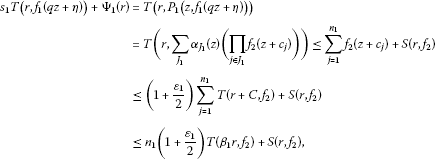(16)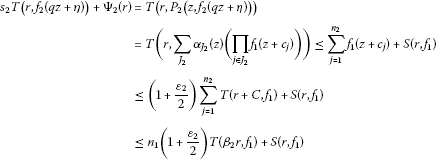(17)

for sufficiently large r and any given ${\beta }_{i}>1$, ${\epsilon }_{i}>0$, $i=1,2$. By Lemma 2.11 and (16), (17), for ${\theta }_{i}=|q|-{\delta }_{i}$ ($0<{\delta }_{i}<|q|$, $0<{\theta }_{i}<1$), $i=1,2$ and sufficiently large r, we get

$\begin{array}{c}{s}_{1}\left(1-{\epsilon }_{1}\right)T\left({\theta }_{1}r,{f}_{1}\right)\le {n}_{1}\left(1+{\epsilon }_{1}\right)T\left({\beta }_{1}r,{f}_{2}\right),\hfill \\ {s}_{2}\left(1-{\epsilon }_{2}\right)T\left({\theta }_{2}r,{f}_{2}\right)\le {n}_{2}\left(1+{\epsilon }_{2}\right)T\left({\beta }_{2}r,{f}_{1}\right),\hfill \end{array}$

outside of a possible exceptional set ${E}_{1}$, ${E}_{2}$ of finite linear measure, respectively. From Lemma 2.12, for any given ${\gamma }_{i}>1$ ($i=1,2$) and sufficiently large r, we can obtain

$\begin{array}{c}{s}_{1}\left(1-{\epsilon }_{1}\right)T\left({\theta }_{1}r,{f}_{1}\right)\le {n}_{1}\left(1+{\epsilon }_{1}\right)T\left({\beta }_{1}{\gamma }_{1}r,{f}_{2}\right),\hfill \\ {s}_{2}\left(1-{\epsilon }_{2}\right)T\left({\theta }_{2}r,{f}_{2}\right)\le {n}_{2}\left(1+{\epsilon }_{2}\right)T\left({\beta }_{2}{\gamma }_{2}r,{f}_{1}\right),\hfill \end{array}$

that is,

$\frac{{s}_{1}\left(1-{\epsilon }_{1}\right)}{{n}_{1}\left(1+{\epsilon }_{1}\right)}T\left(r,{f}_{1}\right)\le T\left(\frac{{\beta }_{1}{\gamma }_{1}}{{\theta }_{1}}r,{f}_{2}\right),\phantom{\rule{2em}{0ex}}\frac{{s}_{2}\left(1-{\epsilon }_{2}\right)}{{n}_{2}\left(1+{\epsilon }_{2}\right)}T\left(r,{f}_{2}\right)\le T\left(\frac{{\beta }_{2}{\gamma }_{2}}{{\theta }_{2}}r,{f}_{1}\right).$
(18)

Case 3.1 Since ${\beta }_{i}>1$, ${\gamma }_{i}>1$, $0<{\theta }_{i}<1$, then we have $\frac{{\beta }_{i}{\gamma }_{i}}{{\theta }_{i}}>1$, $i=1,2$. From (18), and by Lemma 2.10, for any given $\epsilon >0$, there exists a sequence ${r}_{n}\to \mathrm{\infty }$ such that

$\begin{array}{c}\frac{{s}_{1}\left(1-{\epsilon }_{1}\right)}{{n}_{1}\left(1+{\epsilon }_{1}\right)}T\left({r}_{n},{f}_{1}\right)\le {\left(\frac{{\beta }_{1}{\gamma }_{1}}{{\theta }_{1}}\right)}^{\mu \left({f}_{2}\right)+\epsilon }T\left({r}_{n},{f}_{2}\right),\hfill \\ \frac{{s}_{2}\left(1-{\epsilon }_{2}\right)}{{n}_{2}\left(1+{\epsilon }_{2}\right)}T\left({r}_{n},{f}_{2}\right)\le {\left(\frac{{\beta }_{2}{\gamma }_{2}}{{\theta }_{2}}\right)}^{\mu \left({f}_{1}\right)+\epsilon }T\left({r}_{n},{f}_{1}\right)\hfill \end{array}$

for ${r}_{n}>{r}_{0}$. From the above inequalities, we have

$\frac{{s}_{1}\left(1-{\epsilon }_{1}\right)}{{n}_{1}\left(1+{\epsilon }_{1}\right)}\frac{{s}_{2}\left(1-{\epsilon }_{2}\right)}{{n}_{2}\left(1+{\epsilon }_{2}\right)}\le {\left(\frac{{\beta }_{1}{\gamma }_{1}}{{\theta }_{1}}\right)}^{\mu \left({f}_{2}\right)+\epsilon }{\left(\frac{{\beta }_{2}{\gamma }_{2}}{{\theta }_{2}}\right)}^{\mu \left({f}_{1}\right)+\epsilon }.$
(19)

Thus, let ${\epsilon }^{\prime }\to 0$, ${\delta }_{i}\to 0$, ${\beta }_{i}\to 1$ and ${\gamma }_{i}\to 1$ for $i=1,2$ and ${\epsilon }^{\prime }=max\left\{\epsilon ,{\epsilon }_{1},{\epsilon }_{2}\right\}$, since $0<|q|<1$ and ${s}_{1}{s}_{2}\ge {n}_{1}{n}_{2}$, from (19), we can get

$\mu \left({f}_{1}\right)+\mu \left({f}_{2}\right)\ge \frac{log{s}_{1}{s}_{2}-log{n}_{1}{n}_{2}}{-log|q|}.$

Thus, (8) holds.

Case 3.2 Suppose that $|q|>1$. By using the same argument as above, we can get

$\begin{array}{c}{s}_{1}\left(1-{\epsilon }_{1}\right)T\left({\theta }_{1}^{\prime }r,{f}_{1}\right)\le {n}_{1}\left(1+\frac{{\epsilon }_{1}}{2}\right)T\left(r+C,{f}_{2}\right)+S\left(r,{f}_{2}\right),\hfill \\ {s}_{2}\left(1-{\epsilon }_{2}\right)T\left({\theta }_{2}^{\prime }r,{f}_{2}\right)\le {n}_{2}\left(1+\frac{{\epsilon }_{2}}{2}\right)T\left(r+C,{f}_{1}\right)+S\left(r,{f}_{1}\right),\hfill \end{array}$

where ${\theta }_{i}^{\prime }=|q|-{\delta }_{i}$ (${\delta }_{i}>0$ is chosen to be such that ${\theta }_{i}^{\prime }>1$), and r is sufficiently large. We can choose sufficiently small ${\epsilon }_{i}>0$ such that $\frac{1}{{\theta }_{i}^{\prime }}+{\epsilon }_{i}<1$. Thus, it follows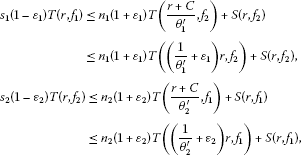outside of a possible exceptional set ${E}_{i}$ ($i=1,2$) of finite logarithmic measure, respectively.

Since ${n}_{1}{n}_{2}\ge {s}_{1}{s}_{2}$, $\frac{1}{{\theta }_{i}^{\prime }}<1$, $i=1,2$, and ${f}_{1}$, ${f}_{2}$ are transcendental, by applying Lemma 3.1 in  and Lemma 2.12, for ${\epsilon }_{i}\to 0$ and ${\delta }_{i}\to 0$, we have

$\rho \left({f}_{1}\right)\le \frac{log{n}_{1}{n}_{2}-log{s}_{1}{s}_{2}}{2log|q|},\phantom{\rule{2em}{0ex}}\rho \left({f}_{2}\right)\le \frac{log{n}_{1}{n}_{2}-log{s}_{1}{s}_{2}}{2log|q|},$

which implies that (9) is true.

Case 3.3 $q=1$ and ${s}_{1}{s}_{2}>{n}_{1}{n}_{2}$. By using the same argument as in Case 3.1, we can get that $\mu \left({f}_{1}\right)+\mu \left({f}_{2}\right)\ge \mathrm{\infty }$.

From Case 3.1, Case 3.2 and Case 3.3, the proof of Theorem 1.9 is completed.

4 Proofs of Theorems 1.7, 1.10 and 1.11

4.1 The proof of Theorem 1.7

Two cases will be considered as follows.

Case 4.1 Suppose that $q=1$ and ${\rho }_{2}\left(f\right)<1$. By applying Lemma 2.1 and Lemma 2.9 for equation (5), we can get

$max\left\{s,t\right\}T\left(r,f\right)\le \left({\mathrm{\Gamma }}_{1}+{\mathrm{\Gamma }}_{2}\right)T\left(r,f\right)+O\left(1\right)+o\left(T\left(r,f\right)\right)$

for all r outside of a possible exceptional set E of finite logarithmic measure. From the above inequality, we have

$max\left\{s,t\right\}\le {\mathrm{\Gamma }}_{1}+{\mathrm{\Gamma }}_{2}+\frac{O\left(1\right)+o\left(T\left(r,f\right)\right)}{T\left(r,f\right)}$

for all r outside of a possible exceptional set E of finite logarithmic measure. Since f is transcendental, we can get

$max\left\{s,t\right\}\le {\mathrm{\Gamma }}_{1}+{\mathrm{\Gamma }}_{2}.$

Case 4.2 Suppose that $q\ne 1$ and $\rho \left(f\right)=0$. By using the same argument as in Case 4.1, we can get

$max\left\{s,t\right\}T\left(r,f\right)\le \left({\mathrm{\Gamma }}_{1}+{\mathrm{\Gamma }}_{2}\right)T\left(r,f\right)+O\left(1\right)+o\left(T\left(r,f\right)\right)$

for all r on a set of logarithmic density 1. Since f is transcendental, from the definition of logarithmic density, we can get

$max\left\{s,t\right\}\le {\mathrm{\Gamma }}_{1}+{\mathrm{\Gamma }}_{2}.$

Thus, this completes the proof of Theorem 1.7.

4.2 The proof of Theorem 1.10

Two cases will be considered to prove Theorem 1.10.

Case 4.3 If $q=1$ and ${\rho }_{2}\left({f}_{1},{f}_{2}\right)<1$. By applying Lemma 2.1 and Lemma 2.9 for the system of complex equations (7), and from the assumptions of Theorem 1.10, we have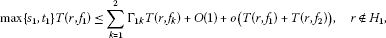(20)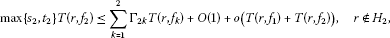(21)

where ${H}_{1}$, ${H}_{2}$ are the sets of finite logarithmic measure. Since $\left({f}_{1},{f}_{2}\right)$ is a transcendental meromorphic solution of (5), from (20) and (21), we have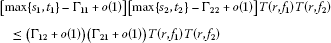(22)

for all r outside of a possible exceptional set ${H}_{3}={H}_{1}\cup {H}_{2}$ of finite logarithmic measure. Thus, from (22), we can get

$\left[max\left\{{s}_{1},{t}_{1}\right\}-{\mathrm{\Gamma }}_{11}\right]\left[max\left\{{s}_{2},{t}_{2}\right\}-{\mathrm{\Gamma }}_{22}\right]\le {\mathrm{\Gamma }}_{12}{\mathrm{\Gamma }}_{21}.$

Case 4.4 Suppose that $q\ne 1$ and $\rho \left({f}_{1},{f}_{2}\right)=0$. By using a similar method as that in Case 4.3, we have

$\begin{array}{c}max\left\{{s}_{1},{t}_{1}\right\}T\left(r,{f}_{1}\right)\le \sum _{k=1}^{2}{\mathrm{\Gamma }}_{1k}T\left(r,{f}_{k}\right)+O\left(1\right)+o\left(T\left(r,{f}_{1}\right)+T\left(r,{f}_{2}\right)\right),\phantom{\rule{1em}{0ex}}r\notin {F}_{1},\hfill \\ max\left\{{s}_{2},{t}_{2}\right\}T\left(r,{f}_{2}\right)\le \sum _{k=1}^{2}{\mathrm{\Gamma }}_{2k}T\left(r,{f}_{k}\right)+O\left(1\right)+o\left(T\left(r,{f}_{1}\right)+T\left(r,{f}_{2}\right)\right),\phantom{\rule{1em}{0ex}}r\notin {F}_{2},\hfill \end{array}$

where ${F}_{1}$, ${F}_{2}$ are the sets of logarithmic density 0. Thus, we get(23)

for all r outside of a possible exceptional set ${F}_{3}={F}_{1}\cup {F}_{2}$ of logarithmic density 0. Thus, from the definition of logarithmic density, we can get

$\left[max\left\{{s}_{1},{t}_{1}\right\}-{\mathrm{\Gamma }}_{11}\right]\left[max\left\{{s}_{2},{t}_{2}\right\}-{\mathrm{\Gamma }}_{22}\right]\le {\mathrm{\Gamma }}_{12}{\mathrm{\Gamma }}_{21}.$

Thus, the proof of Theorem 1.10 is completed.

4.3 The proof of Theorem 1.11

Two cases will be considered as follows.

Case 4.5 If $q=1$ and ${\rho }_{2}\left({f}_{1},{f}_{2}\right)<1$. Since all coefficients $\left\{{a}_{i}^{1}\left(z\right)\right\}$, $\left\{{a}_{i}^{2}\left(z\right)\right\}$, $\left\{{b}_{i}^{1}\left(z\right)\right\}$, $\left\{{b}_{i}^{2}\left(z\right)\right\}$, $\left\{{a}_{\left(i\right)}\left(z\right)\right\}$ and $\left\{{b}_{\left(i\right)}\left(z\right)\right\}$ are not necessarily small functions, by using the same argument as in Lemma 2.9, from Lemma 2.1, we have(24)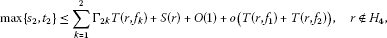(25)

where ${H}_{3}$, ${H}_{4}$ are two sets of finite logarithmic measure, $S\left(r\right)$ is as stated in Section 1.

Suppose that the component ${f}_{1}$ is admissible and ${f}_{2}$ is non-admissible, from (24) we have

$max\left\{{s}_{1},{t}_{1}\right\}\le {\mathrm{\Gamma }}_{11}+o\left(1\right)+{\mathrm{\Gamma }}_{12}\frac{T\left(r,{f}_{2}\right)}{T\left(r,{f}_{1}\right)}+\frac{S\left(r\right)}{T\left(r,{f}_{1}\right)}$

for all r outside of a possible exceptional set of finite logarithmic measure. Thus, from Lemma 2.8, we have

$max\left\{{s}_{1},{t}_{1}\right\}\le {\mathrm{\Gamma }}_{11}$

outside of a possible exceptional set ${E}_{3}={H}_{3}\cup {E}_{2}\cup {E}_{1}$ with finite logarithmic measure, which contradicts the assumptions of Theorem 1.11.

Suppose that the component ${f}_{2}$ is admissible and ${f}_{1}$ is non-admissible, from (25) we have

$max\left\{{s}_{2},{t}_{2}\right\}\le {\mathrm{\Gamma }}_{22}+o\left(1\right)+{\mathrm{\Gamma }}_{21}\frac{T\left(r,{f}_{1}\right)}{T\left(r,{f}_{2}\right)}+\frac{S\left(r\right)}{T\left(r,{f}_{2}\right)}$

for all r outside of a possible exceptional set of finite logarithmic measure. Thus, from Lemma 2.8, we have

$max\left\{{s}_{2},{t}_{2}\right\}\le {\mathrm{\Gamma }}_{22}$

outside of a possible exceptional set ${E}_{4}={H}_{4}\cup {E}_{2}\cup {E}_{1}$ with finite logarithmic measure, which contradicts the assumptions of Theorem 1.11.

Case 4.6 If $q=1$ and $\rho \left({f}_{1},{f}_{2}\right)=0$. By using the same argument as in Case 4.5, noting the definitions of logarithmic measure and logarithmic density, we can prove that the conclusion of Theorem 1.11 is true.

Thus, the proof of Theorem 1.11 is completed.

References

1. 1.

Hayman WK: Meromorphic Functions. Clarendon, Oxford; 1964.

2. 2.

Yang L: Value Distribution Theory. Springer, Berlin; 1993.

3. 3.

Yi HX, Yang CC: Uniqueness Theory of Meromorphic Functions. Kluwer Academic, Dordrecht; 2003. Chinese original: Science Press, Beijing (1995)

4. 4.

Barnett DC, Halburd RG, Korhonen RJ, Morgan W: Nevanlinna theory for the q -difference operator and meromorphic solutions of q -difference equations. Proc. R. Soc. Edinb., Sect. A, Math. 2007, 137: 457–474.

5. 5.

Chen ZX, Huang ZB, Zheng XM: On properties of difference polynomials. Acta Math. Sci., Ser. B 2011, 31(2):627–633.

6. 6.

Chiang YM, Feng SJ:On the Nevanlinna characteristic of $f\left(z+\eta \right)$ and difference equations in the complex plane. Ramanujan J. 2008, 16: 105–129. 10.1007/s11139-007-9101-1

7. 7.

Heittokangas J, Korhonen RJ, Laine I, Rieppo J: Uniqueness of meromorphic functions sharing values with their shifts. Complex Var. Elliptic Equ. 2011, 56: 81–92. 10.1080/17476930903394770

8. 8.

Heittokangas J, Korhonen RJ, Laine I, Rieppo J, Zhang JL: Value sharing results for shifts of meromorphic functions, and sufficient conditions for periodicity. J. Math. Anal. Appl. 2009, 355: 352–363. 10.1016/j.jmaa.2009.01.053

9. 9.

Laine I, Rieppo J, Silvennoinen H: Remarks on complex difference equations. Comput. Methods Funct. Theory 2005, 5(1):77–88.

10. 10.

Laine I, Yang CC: Value distribution of difference polynomials. Proc. Jpn. Acad., Ser. A 2007, 83: 148–151. 10.3792/pjaa.83.148

11. 11.

Liu K, Qi XG: Meromorphic solutions of q -shift difference equations. Ann. Pol. Math. 2011, 101: 215–225. 10.4064/ap101-3-2

12. 12.

Liu K, Yang LZ: Value distribution of the difference operator. Arch. Math. 2009, 92: 270–278. 10.1007/s00013-009-2895-x

13. 13.

Zhang JL, Korhonen RJ:On the Nevanlinna characteristic of $f\left(qz\right)$ and its applications. J. Math. Anal. Appl. 2010, 369: 537–544. 10.1016/j.jmaa.2010.03.038

14. 14.

Halburd RG, Korhonen RJ: Difference analogue of the lemma on the logarithmic derivative with applications to difference equations. J. Math. Anal. Appl. 2006, 314: 477–487. 10.1016/j.jmaa.2005.04.010

15. 15.

Ablowitz MJ, Halburd RG, Herbst B: On the extension of the Painlevé property to difference equations. Nonlinearity 2000, 13: 889–905. 10.1088/0951-7715/13/3/321

16. 16.

Heittokangas J, Korhonen RJ, Laine I, Rieppo J, Tohge K: Complex difference equations of Malmquist type. Comput. Methods Funct. Theory 2001, 1(1):27–39.

17. 17.

Gundersen GG, Heittokangas J, Laine I, Rieppo J, Yang D: Meromorphic solutions of generalized Schröder equations. Aequ. Math. 2002, 63: 110–135. 10.1007/s00010-002-8010-z

18. 18.

Zheng XM, Chen ZX: Some properties of meromorphic solutions of q -difference equations. J. Math. Anal. Appl. 2010, 361: 472–480. 10.1016/j.jmaa.2009.07.009

19. 19.

Gao LY: Systems of complex difference equations of Malmquist type. Acta Math. Sin. 2012, 55: 293–300.

20. 20.

Laine I: Nevanlinna Theory and Complex Differential Equations. de Gruyter, Berlin; 1993.

21. 21.

Halburd, RG, Korhonen, RJ, Tohge, K: Holomorphic curves with shift-invariant hyperplane preimages. arXiv:0903.3236v1

22. 22.

Goldberg AA, Ostrovskii IV Translations of Mathematical Monographs. In Value Distribution of Meromorphic Functions. 2nd edition. Am. Math. Soc., Providence; 2008.

23. 23.

Zhang JL: Value distribution and shared sets of differences of meromorphic functions. J. Math. Anal. Appl. 2010, 367: 401–408. 10.1016/j.jmaa.2010.01.038

24. 24.

Barsegian GA: Gamma Lines: On the Geometry of Real and Complex Functions. Taylor & Francis, London; 2002.

25. 25.

Goldstein R: Some results on factorization of meromorphic functions. J. Lond. Math. Soc. 1971, 4(2):357–364. 10.1112/jlms/s2-4.2.357

26. 26.

Bank S: A general theorem concerning the growth of solutions of first-order algebraic differential equations. Compos. Math. 1972, 25: 61–70.

27. 27.

Gundersen GG: Finite order solutions of second order linear differential equations. Trans. Am. Math. Soc. 1988, 305: 415–429. 10.1090/S0002-9947-1988-0920167-5

Acknowledgements

The authors thank the referee for his/her valuable suggestions to improve the present article. This work was supported by the NSFC (11101201 and 61202313), the Natural Science Foundation of Jiang-Xi Province in China (Grant No. 2010GQS0119, No. 20122BAB201016 and No. 20122BAB201044) and the National Science and Technology Support Plan (2012BAH25F02).

Author information

Authors

Corresponding author

Correspondence to Hong-Yan Xu.

Competing interests

The authors declare that they have no competing interests.

Authors’ contributions

HYX completed the main part of this article, HYX, TBC and BXL corrected the main theorems. All authors read and approved the final manuscript.

Rights and permissions

Reprints and Permissions

Xu, HY., Cao, TB. & Liu, BX. The growth of solutions of systems of complex q-shift difference equations. Adv Differ Equ 2012, 216 (2012). https://doi.org/10.1186/1687-1847-2012-216